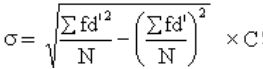# Measures of Dispersion - Online Test

Q1. There is difference or variation among the values. The degree of variation is evaluated by various measures of
Explaination / Solution:
No Explaination.

Q2. A good dispersion should have following characteristics except
Explaination / Solution:

It should be based on all the items so as to be more representative of whole series

Q3. There are two types of measures of dispersion
Explaination / Solution:

Absolute measure of dispersion include range, quartile deviation, standard deviation, variance and relative measure of dispersion include coefficient of range, coefficient of quartile deviation, coefficient of standard deviation, coefficient of variance.

Q4. Following are the Relative measures of dispersion except
Explaination / Solution:

It is a absolute measure of dispersion

Q5. Half of the difference between the first and third quartiles
Explaination / Solution:

It is based on the lower quartile Q1 and the upper quartile Q3. The difference Q3−Q1 is called the inter quartile range. The difference Q3−Q1 divided by 2 is called semi-inter-quartile range or the quartile deviation.

Q6. Standard deviation is also called
Explaination / Solution:

Standard deviation is square root of variance which is also knows as mean square deviation.

Q7. Square of standard deviation is called
Explaination / Solution:

The Standard Deviation is a measure of how spread out numbers are. Its symbol is σ (the greek letter sigma). The formula is easy: it is the square root of the Variance. So the square of σ is variance.

Q8. the positive square-root of the arithmetic mean of the Square of the deviations of the given observation from their arithmetic mean is called
Explaination / Solution:

Standard Deviation is the positive square root of the mean of squared deviations from mean. So if there are five values x1, x2, x3, x4 and x5, first their mean is calculated. Then deviations of the values from mean are calculated. These deviations are then squared. The mean of these squared deviations is the variance. Positive square root of the variance is the standard deviation.

Q9. The average of the squared deviations from the arithmetic mean is called the
Explaination / Solution:

Variance is the expectation of the squared deviation of a random variable from its mean. Informally, it measures how far a set of (random) numbers are spread out from their average value.The variance is the square of the standard deviation, the second central moment of a distribution, and the co variance of the random variable with itself.

Q10. Formula given below is used to calculateExplaination / Solution:

Its a step deviation method to calculate standard deviation.